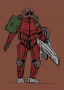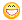About Store Forum Documentation ContactSkeleton Author Message
SebaMemberPost: #16 RE: Skeleton I start to make same test transformation and have one problem to solve. When i move character, the static angle (for example PI/2) didn't change but becouse character move or rotate the transformation is changing. What i have to make to stay in one place when character move, rotate.
08-09-2010 08:52 PM
SebaMemberPost: #17 RE: Skeleton I try solv this problem and nothing work. I have Item with skeleton. I get pos of this point: Quote: if(SkelPoint *point=inv.slot[SLOT_ARM_R]().skeleton->findPoint("secondHand")) { Matrix transform; skelPos=point->pos*inv.slot[SLOT_ARM_R]().matrixScaled(); transform.setPos(skelPos); cskel.transformInWorldSpace(sac.hand_l,transform,true); } skelPos is in good position but hand didn't go to that point. What i have to do? How i should transform Matrix to place hand in good position?
08-11-2010 03:39 PM
EsenthelPost: #18 RE: Skeleton inverse kinematics doesn't work by moving bones, but by rotating them.
08-13-2010 10:07 AM
SebaMemberPost: #19 RE: Skeleton So i working on it but don't move ahead. I try to get angle between bones and then transform arm using that angle. The problem is that when i get position of two bones that are in Player::cskel and get angle when i rotate character nothing change so angle is good. But when i get my SkelPoint form Item::Skeleton and get angle between arm and that point when i rotate character the arm rotate. Why arm rotate in second example? If maybe you have some time you can write some tutorial how to transform character cskel? Code to get matrix: Quote:Matrix3 PLAYER::skelTransform(Vec first, Vec second, Vec dest) { //First - gorna kosc ramienia //Second - dolna kosc ramienia //Dest - punkt docelowy Matrix3 transform; AngleX = AngleBetween(Vec2(first.y,first.z),Vec2(second.y,second.z)); AngleY = AngleBetween(Vec2(first.x,first.z),Vec2(second.x,second.z)); AngleZ = AngleBetween(Vec2(first.x,first.y),Vec2(second.x,second.y)); transform.setRotateXY(AngleX,AngleY); transform.setRotateZ(AngleZ); if(SkelBone *bone=cskel.skeleton()->findBone("ArmLU")) { length=bone->length; length*=1.8; } if(SkelBone *bone1=cskel.skeleton()->findBone("ArmLD")) { length1=bone1->length; length*=1.8; } return transform; } And in void PLAYER::animateUpdateMatrix(C Matrix &body_matrix): Quote: if(inv.slot[SLOT_ARM_R].valid()) // if has item in right hand { if(OrientP *point=cskel.findPoint("HandR")) { Vec current_dir=-point->cross(); // this is the current weapon direction in world space Matrix3 transform; transform.setRotation(current_dir,desired_dir,1); // create matrix which transforms current direction into desired direction cskel.transformInWorldSpace(sac.arm_ru,transform); // call this method to perform a world space transformation } if(OrientP *point=inv.slot[SLOT_ARM_R]().skeleton->findPoint("secondHand")) { if(CSkelBone *bone=cskel.findBone("ArmLU")) if(CSkelBone *bone1=cskel.findBone("ArmLD")) { skelPos=point->pos*inv.slot[SLOT_ARM_R]().matrixScaled(); skelPos1=bone->pos; skelPos2=bone1->pos; Matrix3 transform=skelTransform(skelPos1,skelPos2,skelPos); cskel.transformInWorldSpace(cskel.getBoneI("ArmLD"),transform); } } } Thanks for any advice (This post was last modified: 08-18-2010 10:46 PM by Seba.)
08-18-2010 06:27 PM
EsenthelPost: #20 RE: Skeleton tutorial for advanced inverse kinematics is listed in the version history\roadmap I'll develop it in the future, right now busy with other stuff (iphone/ipad soon)
08-18-2010 07:05 PM
SebaMemberPost: #21 RE: Skeleton Thanks for answer. To keep working i have ask: 1) How get position of skeleton joint between bones? 2) How get length of the bone?
08-18-2010 10:46 PM
EsenthelPost: #22 RE: Skeleton 1) there isn't any joint, there are only bones and their initial position SkelBone::pos * CSkelBone::matrix() 2) SkelBone::length * CSkeleton::scale()
08-18-2010 11:39 PM
SebaMemberPost: #23 RE: Skeleton This commands you post are working good. But how i can get position during animation of mesh. I have something like that: Quote:Matrix skelPos; Skeleton skel; CSkeleton cskel; Quote: if(cskel.findBone("HandR")) { skelPos.setPos(skel.getBone("HandR").pos); skelPos*=cskel.matrix(); } It works good but when i try move this point according to Cskel I can't. I try transform start point using that code: Quote:skelPos.transformAtPos(skel.getBone("HandR").pos*cskel.matrix(),cskel.getBone("HandR").matrix()); skelPos.setTransformAtPos(skel.getBone("HandR").pos*cskel.matrix(),cskel.getBone("HandR").matrix()); But it does't work. Matrix move/rotate according the animation but position is in other place then CSkelBone. I need to get position of CSkelBone during the animation.
12-10-2010 11:07 PM
SebaMemberPost: #24 RE: Skeleton Thanks for all help and reply. It's not my code but something starting to work so thanks for help and answer for my stupid questions. Attached File(s) Image(s)12-19-2010 10:26 PM
 « Next Oldest | Next Newest »